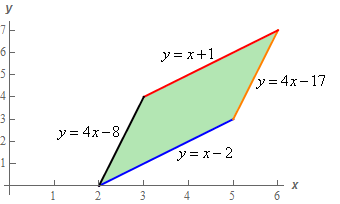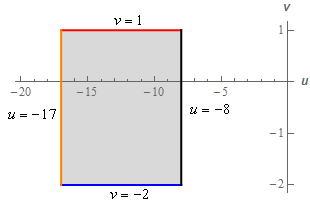Paul's Online Notes
Home / Calculus III / Multiple Integrals / Change of Variables
Show Mobile Notice Show All Notes Hide All Notes
Mobile Notice
You appear to be on a device with a "narrow" screen width (i.e. you are probably on a mobile phone). Due to the nature of the mathematics on this site it is best views in landscape mode. If your device is not in landscape mode many of the equations will run off the side of your device (should be able to scroll to see them) and some of the menu items will be cut off due to the narrow screen width.

### Section 15.8 : Change of Variables

8. Evaluate $$\displaystyle \iint\limits_{R}{{6x - 3y\,dA}}$$ where $$R$$ is the parallelogram with vertices $$\left( {2,0} \right)$$, $$\left( {5,3} \right)$$, $$\left( {6,7} \right)$$ and $$\left( {3,4} \right)$$ using the transformation $$\displaystyle x = \frac{1}{3}\left( {v - u} \right)$$, $$\displaystyle y = \frac{1}{3}\left( {4v - u} \right)$$ to $$R$$.

Show All Steps Hide All Steps

Start Solution

The first thing we need to do is determine the transformation of $$R$$.

First, a sketch of $$R$$.The equations of each of the boundaries of the region are given in the sketch. Given that we know the coordinates of each of the vertices its simple algebra to determine the equation of a line given two points on the line so we’ll leave it to you to verify the equations.

Note that integrating over this region would require three integrals regardless of the order. You can see how each order of integration would require three integrals correct?

Now, let’s transform each of the boundary curves.

$$\displaystyle y = x + 1:\hspace{0.25in}\,\,\,\,\frac{1}{3}\left( {4v - u} \right) = \frac{1}{3}\left( {v - u} \right) + 1\,\,\,\,\,\,\,\,\,\, \to \,\,\,\,\,\,\,3v = 3\,\,\,\hspace{0.25in}\to \,\,\,\,\,\,\,\,\,v = 1$$

$$\displaystyle y = 4x - 17:\hspace{0.25in}\frac{1}{3}\left( {4v - u} \right) = \frac{4}{3}\left( {v - u} \right) - 17\,\,\,\,\,\,\, \to \,\,\,\,\,\,\,3u = - 51\,\,\,\,\,\,\,\, \to \,\,\,\,\,\,\,\,\,u = - 17$$

$$\displaystyle y = x - 2:\hspace{0.25in}\,\,\,\frac{1}{3}\left( {4v - u} \right) = \frac{1}{3}\left( {v - u} \right) - 2\,\,\,\,\,\,\,\,\,\, \to \,\,\,\,\,\,\,3v = - 6\,\,\,\,\,\,\,\,\,\, \to \,\,\,\,\,\,\,\,\,v = - 2$$

$$\displaystyle y = 4x - 8:\hspace{0.25in}\frac{1}{3}\left( {4v - u} \right) = \frac{4}{3}\left( {v - u} \right) - 8\hspace{0.25in}\to \,\,\,\,\,\,\,3u = - 24\,\,\,\,\,\,\, \to \,\,\,\,\,\,\,\,\,u = - 8$$

Here is a sketch of the transformed region and note that the transformed region will be much easier to integrate over than the original region.So, the limits for the transformed region are,

$\begin{array}{c} - 17 \le u \le - 8\\ \, - 2 \le v \le 1\end{array}$

Note as well that this is going to be a much nice region to integrate over than the original region.

Show Step 2

We’ll need the Jacobian of this transformation next.

$\frac{{\partial \left( {x,y} \right)}}{{\partial \left( {u,v} \right)}} = \left| {\begin{array}{*{20}{c}}\frac{{\partial x}}{{\partial u}}}&\displaystyle {\frac{{\partial x}}{{\partial v}}}\\\displaystyle {\frac{{\partial y}}{{\partial u}}}&\displaystyle {\frac{{\partial y}}{{\partial v}}}\end{array}} \right| = \left| {\begin{array}{*{20}{c}}{ \displaystyle - \frac{1}{3}}&\frac{1}{3}}\\- \frac{1}{3}}&\frac{4}{3}}\end{array}} \right| = - \frac{4}{9} - \left( { - \frac{1}{9}} \right) = - \frac{1}{3$ Show Step 3

We can now write the integral in terms of the “new” $$uv$$ coordinates system.

\begin{align*}\iint\limits_{R}{{6x - 3y\,dA}} & = \int_{{ - 17}}^{{ - 8}}{{\int_{{ - 2}}^{1}{{\left[ {6\left( {\frac{1}{3}} \right)\left( {v - u} \right) - 3\left( {\frac{1}{3}} \right)\left( {4v - u} \right)} \right]\left| { - \frac{1}{3}} \right|\,dv}}\,du}}\\ & = \int_{{ - 17}}^{{ - 8}}{{\int_{{ - 2}}^{1}{{ - \frac{1}{3}\left( {2v + u} \right)\,dv}}\,du}}\end{align*}

Don’t forget to add in the Jacobian and don’t forget that we need absolute value bars on it. In this case we can just drop the absolute value bars by also dropping the minus sign since we just have a number in the absolute value.

Also, the simplified integrand didn’t suggest any one order of integration over the other and so we just chose one to work with. The other order would be just as easy to have worked with.

Show Step 4

Finally, let’s evaluate the integral.

\begin{align*}\iint\limits_{R}{{6x - 3y\,dA}} & = \int_{{ - 17}}^{{ - 8}}{{\int_{{ - 2}}^{1}{{ - \frac{1}{3}\left( {2v + u} \right)\,dv}}\,du}}\\ & = \int_{{ - 17}}^{{ - 8}}{{\left. {\left( { - \frac{1}{3}\left( {{v^2} + uv} \right)} \right)} \right|_{ - 2}^1\,du}}\\ & = \int_{{ - 17}}^{{ - 8}}{{1 - u\,du}}\\ & = \left. {\left( {u - \frac{1}{2}{u^2}} \right)} \right|_{ - 17}^{ - 8}\\ & = \require{bbox} \bbox[2pt,border:1px solid black]{{\frac{{243}}{2}}}\end{align*}